﻿ A New Tipe of Contact Potential Difference and Electrification of Superconducting Coils and Tori

### A New Tipe of Contact Potential Difference and Electrification of Superconducting Coils and Tori

F. F. MendeOPEN ACCESSPEER-REVIEWED

## A New Tipe of Contact Potential Difference and Electrification of Superconducting Coils and Tori

B.I. Verkin Institute for Low Temperature Physics and Engineering NAS, Ukraine

### Abstract

In the article is examined new physical phenomenon the electrocurent contact potential difference, whose value depends on the current, which flows along the conductor. Unfortunately, the computed values of electrocurent contact a potential difference proved to be considerably lower than the potentials, observed in the experiment. The carried out experiments and calculations showed that the discovered electrization of the superconductive windings and tori finds its explanation in the concept of scalar-vector potential, developed by the author. This concept assumes the dependence of the scalar potential of charge on its relative speed.

### At a glance: Figures

1234
Prev Next

• Mende, F. F.. "A New Tipe of Contact Potential Difference and Electrification of Superconducting Coils and Tori." American Journal of Electrical and Electronic Engineering 2.5 (2014): 146-151.
• Mende, F. F. (2014). A New Tipe of Contact Potential Difference and Electrification of Superconducting Coils and Tori. American Journal of Electrical and Electronic Engineering, 2(5), 146-151.
• Mende, F. F.. "A New Tipe of Contact Potential Difference and Electrification of Superconducting Coils and Tori." American Journal of Electrical and Electronic Engineering 2, no. 5 (2014): 146-151.

 Import into BibTeX Import into EndNote Import into RefMan Import into RefWorks

### 1. Electrocurent Contact Potential Difference

The contact potential difference this is the potential difference, which appears between the located in the electrical contact conductors under the thermodynamic equilibrium conditions. As a result this between the conductors occurs the electron transfer until the Fermi levels in both conductors are made even. The established contact potential difference is equal to difference the work function of conductors, referred to the electron charge. But from the attention of researchers slipped off still one type of contact potential difference, which occurs with the flow of the current through the superconductors.

The amount of the ponderomotive force gradient, which acts on the single square of the surface of conductor is determined by the relationshipwhereis magnetic field on the surface of conductor,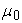is magnetic permeability.

This force is applied to the moving electrons and attempts to press electronic flux. In order to balance the force indicated, near the surface of superconductor is formed the positively charged layer, depleted by electrons the electrostatic field of this layer it balances the ponderomotive force (Figure 1)

If the superconductor, along which flows the current, to lead into the contact with the normal metal, then the part of the electrons from this metal will pass to depletion layer and between the superconductor and the normal metal is formed the contact potential difference, which is proportional to the square of current. For forming the layer, depleted by electrons, the energy of magnetic field is expended, and for enumerating the contact potential difference should be made level energy of the magnetic field R of electrostatic energy of depletion layer.

Figure 1. Compression of the electronic flux, which flows along the conductor

A contact potential difference comprises for the case of round conductor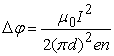(1.1)

### 2. Experimental Study of the Electrization of the Superconductive Windings and Tori

For the introduction of current into the superconductive winding was used the transformer with the iron core, cooled to helium temperatures using as the secondary winding of transformer the superconductive winding, connected with the external superconductive outline, it is possible without the presence of galvanic contacts to introduce current into this outline. For the purpose of the decrease of the inductance of outer duct it is executed in the form double winding (further noninductive windings). In the transformer was used ring-shaped core made of transformer steel with a cross section 9 cm2. The primary and secondary windings of transformer were wound by niobium-titanium wire with the copper coating and contained 150 and 10 turns respectively. Thus, transformer has a transformation ratio 15. The wire diameter composed 0.25 mm. The secondary winding of transformer is connected in series with the solenoid with the small inductance, which is wound bifilar and contains 2448 turns of the same wire. The overall length of coil composes 910 m. The ends of solenoid and secondary winding of transformer are welded with the aid of the laser welding. Noninductive windings is wound on the body from teflon resin. Inside and outside diameter of the winding of solenoid 35 and 90 mm respectively, the width of the coil 30 mm. To the midpoint of noninductive windings is connected the central core of the coaxial, which emerges outside cryostat, the same coaxial is connected also to the screen, which surrounds noninductive windings. The construction of noninductive windings and elements of its fastening is shown in Figure 2

By numbers in the figure are designated the following elements: 1- aluminum body, 2 - teflon bushing, 3 - teflon disk, 4- clamp, 5 - counter, 6- bolt, 7- copper screen, 8 - teflon body. Noninductive windings is wound on teflon body 8, which is concluded in aluminum body 1. Outside solenoid is surrounded by copper screen 7. To body 1 with the aid of bolt 6 and teflon bushing 2 is fastened teflon disk 3, on which is installed clamp 4. The turns of the secondary winding of transformer cover clamp 4, through which, without concerning it, is passed the magnetic circuit of transformer. Entire construction is attached to the transformer by means of counters 5. Transformer together with the noninductive windings is placed in the tank of helium cryostat.

The diagram of the connection of coaxials to noninductive windings and to screen to its surrounding is shown in Figure 3.

By the figure are accepted the following designations: 1- noninductive windings, 2 - the screen of noninductive windings, 3,4 - coaxials, 5 - the common screen, which the helium tank is. Resistance between the grounded elements, the screen of solenoid and solenoid itself composes not less than ~1014 Ohm. The elements, utilized in the construction, had the following capacities relative to the earth: coaxial 3 - 44 pF, coaxial 4 - 27 pF, capacity screen - the earth it comprises - 34 pF, capacity screen - solenoid compose - 45 pF, as the electrometer was used by capacitive vibrating reed electrometer with a input capacitance 60 pF and a input resistance ~1014 Ohm.

With the measurements the electrometer was connected to the solenoid with the aid of coaxial 3, and screen 2 with the aid of the coaxial I was grounded. Current into the primary winding of transformer was introduced from the source of direct current, indication of electrometer in this case they did not depend on direction of flow. With the strengths of introduced current ~9 A occurred the discharge of the indications of electrometer. This means that the current in the winding of solenoid reached its critical value, and winding converted to normal state.

The experimental dependence of a contact potential difference is shown in Figure 4. The values of a voltage drop across figure shows with the opposite sign.

Experimental data are given in the Table 1.

#### Table 1.Download asPowerPoint Slide

Veiw figureView current table in a new window

In the first graph of table are given the value of the current, introduced into the primary winding. In the second graph are given the values of the current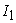in the noninductive windings calculated on the basis of the value of the transformation ratio of equal to 15. In this case it is assumed that in entire range of the introduced currents the magnetization of core remains proportional to current. In the third graph are given the values of magnetic pour on the surface of the superconductive wires of noninductive windings. In the fourth graph the indications of electrometer are indicated. In the fifth graph the indications of the effective values of a potential difference are indicated. These values correspond to the value of potential between the solenoid and the screen to the connection to the screen of the total capacitance of coaxial and electrometer. In the sixth graph the indications of the coefficient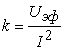, which indicates the deviation of the obtained dependence on the quadratic law. The root-mean-square relative deflection of the coefficient of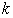from its average value equal to 1.93 composes 0.13, which gives relative root-mean-square error 7%. Thus, the obtained dependence between the current and the measured value of potential is very close to the quadratic law. It is also evident from the table that with the values of current in the conductors of solenoid on the order 120 A, the field strength on their surface reaches its critical value, which for the utilized superconductor composes 1.5x105 A/m, with which and is connected the discharge of the indications of electrometer with reaching of these currents. With this is connected the discharge of the indications of the electrometer, which occurs upon transfer of the superconductive winding into the normal state and it leads to damping of the superconductive current.

The measurement of a potential difference was conducted also according to the diagram, when electrometer was connected to the screen with the aid of coaxial 4, but noninductive windings anywhere was not connected. In this case was obtained the dependence analogous to that, which was depicted in Figure 4, but the amplitude of stress was approximately two times less. It is interesting to note that the dependence indicated remains even when noninductive windings it is grounded. This it indicates that, the electric fields, which induce potential in the screen, are created by the charges, which move into noninductive windings. These results cannot explain the existing laws of electrodynamics, since. the obtained result indicates that the charge is not the invariant of speed.

For the first time communication about the electrization of the superconductive windings, into which was introduced direct current, was published in article . Subsequently similar studies were executed by the authors of this article [4, 5, 6, 7, 8, 9, 10].

Important circumstance is the fact that with potential measurement of screen 1, in which are placed noninductive windings, variation, given in Figure 4 are repeated. Similar results were obtained also in the experiments with the niobium superconductive torus. the diagram of experiment is shown in Figure 5. Inside the conducting screen was placed the second conducting screen, in which the superconductive torus, made from niobium, was located, and electrometer was connected by these screens. In the experiment, as external screen 1, the yoke of transformer, made from transformer steel, was used. On the central rod of yoke was located primary winding with 2, wound by niobium-titanium wire and which contains 1860 turns. Torus-shaped metal screen 3, made from copper, was located on the same rod. Torus 4, made from niobium, was located inside this screen. The outer diameter of niobium torus was 76 mm, and internal 49 mm. Transformer was placed in the tank of helium cryostat and was cooled to the helium temperature, in this case the yoke of transformer and helium tank were grounded. The current was induced during the introduction of direct current into the primary winding of transformer in the superconductive torus, and electrometer fixed the appearance between screen 3 and yoke of transformer a potential difference. This means that the niobium torus, located inside screen 3 during the introduction into it of direct current ceases to be electrically neutral. The constant value current in the superconductive torus 1860 times exceeds the current, introduced into the primary winding of transformer.

The dependence of a potential differenceon the current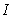, introduced into the primary winding of transformer, it is shown in Figure 6.

Figure 6. Dependence of a potential difference boundary by screen 3 by the yoke of transformer on the current, introduced into the primary winding of transformer

The obtained values of a potential difference, in comparison with the case of the superconductive wire winding, proved to be considerably smaller, this is connected with the considerably smaller surface of torus, in comparison with the surface of wire winding. This is connected with the fact that the surface of torus considerably less than the surface of the wire of solenoid. The form of the dependence of a potential difference on the introduced current also strongly differs. Quadratic section is observed only in the very small initial section up to the values of currents ~2 A, introduced into the primary winding. Further this dependence becomes practically linear with the small slope angle. The discharge of the indications of electrometer it was not observed.

In the case of noninductive windings the superconductive current is evenly distributed over the surface of wire and reaches its critical value in all its sections simultaneously. With this is connected the simultaneous passage of the entire winding of solenoid into the normal state, with the reaching in the wire of the critical value of current.

In the case of torus the process of establishing the superconductive current on its surface occurs differently. That introduced into the direct current superconducting torus is very unevenly distributed over its surface. Maximum current densities occur on the internal surface of torus, and they are considerably less on the periphery. With this is connected the fact that the internal surfaces of torus begin to convert to normal state earlier than external. The process of passing the torus into the normal state normal phase begins to be moved from the interior of torus to the external regions. Process lasts until entire torus passes into the normal state. But why in this case up to the moment of passing the torus into the normal state does not occur the discharge of current, as it takes place in the case of wire solenoid? This niobium is connected with the fact that the superconductor of the second kind, and he has the sufficiently significant region of magnetic pour on, with which it is in the mixed state. In this case inside the massive superconductor Abrikosov's vortices penetrate. The circumstance that the indications of electrometer do not have a discharge of indications, he indicates the fact that superconductive torus it is in the mixed state. In this case vortex structures also present the superconductive currents and they have an effect on the electrization of torus.

If we change direction of flow in the primary winding, then the dependence, similar to that depicted in Figure 6, is repeated, however, it is observed strong hysteresis. This is connected with the fact that the vortices, which penetrated into the depths of the superconductor, they are attached on the stacking faults, falling into potential wells, that also leads to hysteresis.

The electrization of the superconductive windings and tori does not find the explanation of the within the framework existing electrodynamics, these results do not find explanation and within the framework the special theory of relativity., Is the thus far only theory, which is capable of explaining the obtained results, the concept of scalar-vector potential, which assumes within the framework the conversions of Galileo the dependence of the scalar potential of charge on his speed [4, 7, 8, 9, 10].

### 3. Results of other Authors and the Consideration of the Obtained Results

The thorough the study of the electrization of the superconductive windings is carried out in work . In this case also was used the double winding (noninductive windings) from the niobium-titanium wire, copper-plated. The length of wire is 701 m, and the diameter of wire is equal to 0,128 mm. The schematic of experimental installation is shown in Figure 7, and its composition and elements are shown in Figure 8.

In the installation besides mechanical keys is located the thermal key S4, which makes it possible to convert the part of the superconductive locked outline to normal state. In the chain of outline is a resistance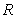, by which is shunted noninductive windings and solenoid. Solenoid has a small quantity of turns and is used for measuring the current into noninductive windings. Measurement is conducted with the aid of the Hall pickup, which measures the magnetic field of solenoid. The resistance of the thermal key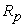is selected in such a way that would be satisfied the condition. With satisfaction of this condition after the switching on of thermal key the current of noninductive windings flows through the resistance of. This gives possibility with the aid of the electrometer, connected to the outline to observe the behavior of potential on the outline in the process of damping current into noninductive windings. Outline and thermal key are located in the brass screen. Inside the screen to teflon counter are fastened the contacts, through which is introduced the current into noninductive windings. These contacts are fastened to beryllium- Cooper springs, to the contacts are connected the wires of noninductive windings. Current to the contacts brings with the aid of current of rod. When current of rod raises upward, contacts are opened.

In the process of conducting the experiments were realized two operating modes.

In the first regime current was introduced into the outline, thermal key and then was included. In this regime occurred the exponential damping of current into noninductive windings, and the behavior of electric potential on the outline was observed with the aid of the electrometer.

The obtained dependences are represented in Figure 9.

From the represented dependences is evident that to the great significances of current correspond the high absolute values of potentials, which coincides with the results of the experiments, represented in Figure 4. The amplitude of potential change composes ~10 mV. Dependence between the potential and the current into noninductive windings corresponds to quadratic law.

The special interest the results of the experiment, when electrometer was connected directly to bras of shields (Faraday Cage), present, but outline is not anywhere connected. This experiment is described in the division B. Variation II. For the realization of this experiment from the composition of outline thermal key was excluded. Current into noninductive windings was introduced with the aid of current of rod, which after the introduction of current rose upward and current source was disconnected from the outline. At the same moment to bras of shields was connected the electrometer. The diagram of this experiment is represented in Figure 10.

The experimental results of experiment are represented in Figure 11.

Is evident that a change of the current into noninductive windings leads to the appearance of potential on the outline, which is located into Faraday Cage. This result contradicts the existing laws of electrodynamics, since gives the foundation for assuming that the charge is not the invariant of speed. Similar results were obtained both by contributor in the experiments with the superconductive windings and by tori, described in the previous division.

Figure 11. Experimental results, obtained according to the diagram Variation II

The thus far only theory, which can explain the phenomena indicated, is the concept of scalar- vector potential developed by the author, in which the scalar potential of charge depends on its speed [4, 7, 8, 9, 10].

This concept assumes that the scalar potential is determined by the relationshipwhere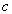is speed of light,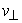is normal component of the electron velocity to the vector, which connects the moving charge and the observation point,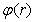is scalar potential of fixed charge.

The electric field of the moving electron will be determined by the relationship:If is located the plane layer of the electrons, which move with the speed of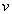, whose thickness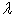, then the value of the electric field, normal to the surface of layer, will comprise: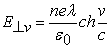whereis the electric field of fixed bed,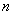is electron density.

In the metal, this field is compensated by the electric field of lattice. Consequently, increase in the electric field on the surface of metallic layer will compose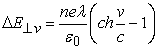Decomposing hyperbolic cosine in a number and leaving only first two terms of expansion, we obtain(3.1)

The value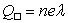determines the specific charge of the layer, and the value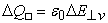(3.2)

determines the increase in the specific charge of surface layer, caused by electron motion. Comparing relationships (3.1) and (3.2), we obtain increase in the specific charge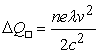(3.3)

In the superconductor the depth of penetration of current is equal to the London depth of penetration, therefore this relationship applies to superconductors, if we consider that the speed of electron motion in the surface layer is equal.

The speed of electron motion in the surface layer of superconductor is connected with the magnetic field with the relationship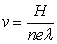(3.4)

Substituting relationship (3.4) in relationship (3.3), we obtain: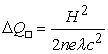(3.5)

The magnetic field on its surface of superconductor, equal to specific current, can be determined from the relationship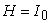(3.6)

If along the round superconductor, whose diameter is equal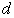, flows the currentand its depth of penetration is equal, then specific current is determined from the relationship(3.7)

Taking into account relationships (3.6) and (3.7) from relationship (3.5) we obtain:In order to obtain total increase in the charge of the surface in question, should be this relationship multiplied by the surface area. Since the surface area of round conductor is connected with its length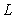and diameter with the relationship, finally we obtain: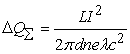If we with the aid of this charge load capacitor with the capacity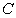, then a voltage drop across the terminals of capacitor will be equal(3.8)

Is obtained quadratic dependence between the current, which flows through the superconductive wire and the potential, that also is observed in the experiments examined. Into this relationship enter known tabular values, parameters of chain and current, which flows through the superconductive winding. If this outline was placed into the metal screen, then the electric fields of winding will direct on this screen potential.

In the first division was obtained relationship (1.1), which determines a electrotocurent potential difference, but calculations showed that the value of this voltage drop across three orders is lower than the value of the potentials, obtained in the experiment. Therefore the potential difference indicated it is possible not to consider.

Relationship (3.8) explains quadratic dependence between the potential and the current, introduced into noninductive windings by current. However, absolute values of potentials calculated with the aid of this relationship prove to be several times greater than observed in the experiment.

Such divergences are connected with the fact that with p ofofri calculations we they considered that the electric fields form the charges of the flat surface of unconfined in the sizes. This means that such fields are uniform along the plane and their value does not depend from the distance to the surface. In actuality the annular conductors of different diameters form electric fields; therefore their electric fields strongly depend on distance from these rings, with this are connected the existing divergences.

### 4. Conclusion

In the article is examined new physical phenomenon the electrotocurent contact potential difference, whose value depends on the current, which flows along the conductor. Unfortunately, the computed values of electrotocurent contact a potential difference proved to be considerably lower than the potentials, observed in the experiment. The carried out experiments and calculations showed that the discovered electrization of the superconductive windings and tori finds its explanation in the concept of scalar- vector potential, developed by the author. This concept assumes the dependence of the scalar potential of charge on its relative speed.

### Reference

  W. F. Edwards, C.S. Kenyon, D.K. Lemon, Continuing investigation into possible electric arising from steady conduction current, Phys. Rev. D 14, 922, 1976.In article CrossRef  Roser W.G.V. Second-Order Electric Field due to a Conducting Curent. American Journal of Physics, 1962, v. 30, №7.In article  Don A. Baker. Second-Order Electric Field due to a Conducting Curent. American Journal of Physics, 1964, v. 32, № 2.In article  F. F. Mende, On refinement of equations of electromagnetic induction, Kharkov, deposited in VINITI, No 774-B88 Dep., 1988.In article  F.F. Mende, On secondary electric fields excited at direct currents flowing through superconductors. Kharkov, deposited in VINITI 05.11.92. No. 3182-B92. Dep., 1992.In article  F. F. Mende, Experimental corroboration and theoretical interpretation of dependence of charge velocity on DC flow velocity through superconductors. Proceedings International Conference “Physics in Ukraine”, Kiev 22-27 June 1993.In article  F. F. Mende, Are there errors in modern physics. Kharkov, Constant, 2003.In article  F. F. Mende, On refinement of certain laws of classical electrodynamics, arXiv.org/abs/physics/0402084.In article  Mende F. F. Conception of the scalar-vector potential in contemporary electrodynamics, arXiv.org/abs/physics/0506083.In article  F. F. Mende, New approachesin contemporary classical electrodynamics. Part II, Engineering Physics, №2, 2013.In article